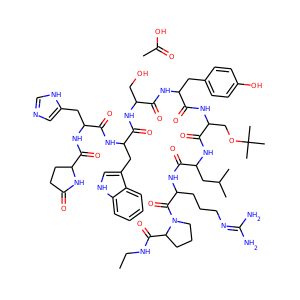877-202-0205     support@chemchart.com     @chemchartBuserelin acetate
Weight 1299.499 g/mol C62H90N16O15 16 16 4 32

# Buserelin acetate (68630-75-1)

"What do you need help with?"### Alternate Names

• SMILES
CCNC(=O)C1CCCN1C(=O)C(CCCN=C(N)N)NC(=O)C(CC(C)C)NC(=O)C(COC(C)(C)C)NC(=O)C(CC2=CC=C(C=C2)O)NC(=O)C(CO)NC(=O)C(CC3=CNC4=CC=CC=C43)NC(=O)C(CC5=CN=CN5)NC(=O)C6CCC(=O)N6.CC(=O)O
• InChIKey
PYMDEDHDQYLBRT-UHFFFAOYSA-N